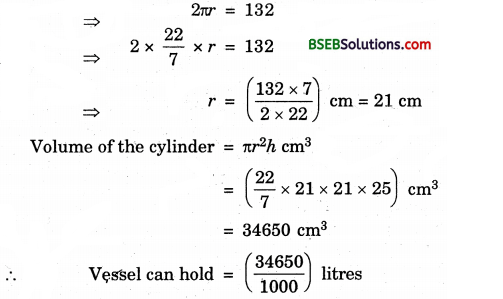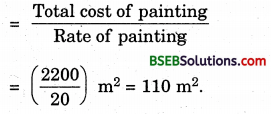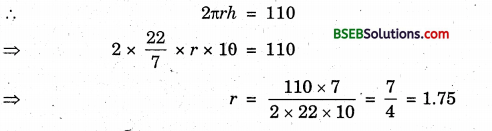# HSSlive: Plus One & Plus Two Notes & Solutions for Kerala State Board

## BSEB Class 9 Maths Chapter 13 Surface Areas and Volumes Ex 13.6 Textbook Solutions PDF: Download Bihar Board STD 9th Maths Chapter 13 Surface Areas and Volumes Ex 13.6 Book AnswersBSEB Class 9 Maths Chapter 13 Surface Areas and Volumes Ex 13.6 Textbook Solutions PDF: Download Bihar Board STD 9th Maths Chapter 13 Surface Areas and Volumes Ex 13.6 Book Answers

BSEB Class 9th Maths Chapter 13 Surface Areas and Volumes Ex 13.6 Textbooks Solutions and answers for students are now available in pdf format. Bihar Board Class 9th Maths Chapter 13 Surface Areas and Volumes Ex 13.6 Book answers and solutions are one of the most important study materials for any student. The Bihar Board Class 9th Maths Chapter 13 Surface Areas and Volumes Ex 13.6 books are published by the Bihar Board Publishers. These Bihar Board Class 9th Maths Chapter 13 Surface Areas and Volumes Ex 13.6 textbooks are prepared by a group of expert faculty members. Students can download these BSEB STD 9th Maths Chapter 13 Surface Areas and Volumes Ex 13.6 book solutions pdf online from this page.

## Bihar Board Class 9th Maths Chapter 13 Surface Areas and Volumes Ex 13.6 Books Solutions

 Board BSEB Materials Textbook Solutions/Guide Format DOC/PDF Class 9th Subject Maths Chapter 13 Surface Areas and Volumes Ex 13.6 Chapters All Provider Hsslive

## How to download Bihar Board Class 9th Maths Chapter 13 Surface Areas and Volumes Ex 13.6 Textbook Solutions Answers PDF Online?

2. Click on the Bihar Board Class 9th Maths Chapter 13 Surface Areas and Volumes Ex 13.6 Answers.
3. Look for your Bihar Board STD 9th Maths Chapter 13 Surface Areas and Volumes Ex 13.6 Textbooks PDF.
4. Now download or read the Bihar Board Class 9th Maths Chapter 13 Surface Areas and Volumes Ex 13.6 Textbook Solutions for PDF Free.

## BSEB Class 9th Maths Chapter 13 Surface Areas and Volumes Ex 13.6 Textbooks Solutions with Answer PDF Download

Find below the list of all BSEB Class 9th Maths Chapter 13 Surface Areas and Volumes Ex 13.6 Textbook Solutions for PDF’s for you to download and prepare for the upcoming exams:

## BSEB Bihar Board Class 9th Maths Solutions Chapter 13 Surface Areas and Volumes Ex 13.6

Question 1.
The circumference of the base of a cylindrical vessel is 132 cm and its height is 25 cm. How many litres of water can it hold ? (1000 cm³ = 1l)
Solution:
Let r cm be the radius of the base and h cm be the height of the cylinder.
Circumference of the base = 132 cmi.e., 34.65 litres of water.

Question 2.
The inner diameter of a cylindrical wooden pipe is 24 cm and its outer diameter is 28 cm. The length of the pipe is 35 cm. Find the mass of the pipe, if 1 cm³ of wood has a mass of 0.6 g.
Solution:
We have h = Height of the cylindrical pipe = 35 cm
R = External radius = (282) cm = 14 cm
r = Internal radius = (242) cm = 12 cm
Volume of the wood used in making the pipe = Volume of the external cylinder – Volume of the internal cylinder
= πR²h – πr²h = π(R² – r²)h
= 227 x (14² – 12²) x 35 cm³
= 227 x 26 x 2 x 35 cm³
= 5720 cm³
Weight of 1 cm³ = 0.6 g
∴ Weight of 5720 cm³ = (5720 x 0.6) g
= (5720×0.61000) kg
= 3.432 kg

Question 3.
A soft drink is available in two packs – (i) a tin can with a rectangular base of length 5 cm and width 4 cm, having a height of 15 cm and (ii) a plastic cylinder with circular base of diameter 7 cm and height 10 cm. Which container has greater capacity and by how much?
Solution:
(i) Capacity of tin can = lbh cm³
= (5 x 4 x 15) cm³
= 300 cm³

(ii) Capacity of plastic cylinder = πr²h cm³
= (227 x 72 x 72 x 10) cm
= 385 cm³
Thus, the plastic cylinder has greater capacity by 85 cm³.

Question 4.
If the lateral surface of a cylinder is 94.2 cm² and its height is 5 cm, then find (i) radius of its base (ii) its volume (Use π = 3.14)
Solution:
(i) Let r be the radius of the base and h be the height of the cylinder. Then,
Lateral surface = 94.2 cm²
⇒ 2 πrh = 94.2
⇒ 2 x 3.14 x r x 5 = 94.2
⇒ r = 94.22×3.14×5 = 3
Thus, the radius of its base = 3 cm.

(ii) Volume of the cylinder = πr²h
= (3.14 x 32 x 5) cm³
= 141.3 cm³.

Question 5.
It costs Rs 2200 to paint the inner curved surface of a cylindrical vessel 10 m deep. If the cost of paintin g is at the rate of Rs 20 per m², find
(i) inner curved surface area of the vessel.
(iii) capacity of the vessel.
Solution:
(i) Inner curved surface area of the vessel(ii) Let r be the radius of the base and h be the height of the cylindrical vessel.Thus, the radius of the base = 1.75 m.

(iii) Capacity of the vessel = πr²h
= (227 x 74 x 74 x 10) m³
= 96.25 m³

Question 6.
The capacity of a closed cylindrical vessel of height 1 m is 15.4 litres. How many square metres of metal sheet would be needed to make it?
Solution:
Capacity of a closed cylindrical vessel = 15.4 litres
= (15.4 x 11000) m³ = 0.0154 m³
Let r be the radius of the base and h be the height of the vessel. Then,
Volume = πr²h = πr² x 1 = πr² [∵ h = 1 m]
πr² = 0.0154
⇒ 227 x r² = 0.0154
⇒ r² = 0.0154×722 = 0.0049
⇒ r = 0.0049‾‾‾‾‾‾√ = 0.07
Thus, the radius of the base of vessel = 0.07 m Metal sheet needed to make the vessel
= Total surface area of the vessel
= 2πrh + 2πr² = 2πr(h + r)
= 2 x 227 x 0.07 x (1 + 0.07) m²
= 44 x 0.01 x 1.07 m²
= 0.4708 m².

Question 7.
A lead pencil consists of a cylinder of wood with a solid cylinder of graphite filled in the interior. The diameter of the pencil is 7 mm and the diameter of the graphite is 1 mm. If the length of the pencil is 14 cm, find the volume of the wood and that of the graphite.
Solution:
Diameter of the graphite cylinder = 1 mm = 110 cm
Length of the graphite = 14 cm
Volume of the graphite cylinder = (227 x 120 x 120 x 14) cm³
Diameter of the pencil = 7 mm = 710 cm = 0.11 cm³
∴ Radius of the pencil = 720 cm
and, length of the pencil = 14 cm
∴ Volume of the pencil = (227 x 720 x 720 x 14) cm³
= 5.39 cm³
Volume of wood = Volume of the pencil – Volume of the graphite
= (5.39 – 0.11) cm³
= 5.28 cm³.

Question 8.
A patient in a hospital is given soup daily in a cylindrical bowl ofL diameter 7 cm. If the bowl is filled with soup to a height of 4 cm, how much soup the hospital has to prepare daily to serve 250 patients ?
Solution:
Diameter of the cylindrical bowl = 7 cm
Height of serving bowl = 4 cm
∴ Soup saved in on serving = Volume of the bowl
= πr²h
= (227 x 72 x 72 x 4) cm³
= 1.54 cm³
Soup-served to 250 patients = (250 x 1.54) cm³
= 38500 cm³
i.e., 38.5l.

## Bihar Board Class 9th Maths Chapter 13 Surface Areas and Volumes Ex 13.6 Textbooks for Exam Preparations

Bihar Board Class 9th Maths Chapter 13 Surface Areas and Volumes Ex 13.6 Textbook Solutions can be of great help in your Bihar Board Class 9th Maths Chapter 13 Surface Areas and Volumes Ex 13.6 exam preparation. The BSEB STD 9th Maths Chapter 13 Surface Areas and Volumes Ex 13.6 Textbooks study material, used with the English medium textbooks, can help you complete the entire Class 9th Maths Chapter 13 Surface Areas and Volumes Ex 13.6 Books State Board syllabus with maximum efficiency.

## FAQs Regarding Bihar Board Class 9th Maths Chapter 13 Surface Areas and Volumes Ex 13.6 Textbook Solutions

#### How to get BSEB Class 9th Maths Chapter 13 Surface Areas and Volumes Ex 13.6 Textbook Answers??

Students can download the Bihar Board Class 9 Maths Chapter 13 Surface Areas and Volumes Ex 13.6 Answers PDF from the links provided above.

#### Can we get a Bihar Board Book PDF for all Classes?

Yes you can get Bihar Board Text Book PDF for all classes using the links provided in the above article.

## Important Terms

Bihar Board Class 9th Maths Chapter 13 Surface Areas and Volumes Ex 13.6, BSEB Class 9th Maths Chapter 13 Surface Areas and Volumes Ex 13.6 Textbooks, Bihar Board Class 9th Maths Chapter 13 Surface Areas and Volumes Ex 13.6, Bihar Board Class 9th Maths Chapter 13 Surface Areas and Volumes Ex 13.6 Textbook solutions, BSEB Class 9th Maths Chapter 13 Surface Areas and Volumes Ex 13.6 Textbooks Solutions, Bihar Board STD 9th Maths Chapter 13 Surface Areas and Volumes Ex 13.6, BSEB STD 9th Maths Chapter 13 Surface Areas and Volumes Ex 13.6 Textbooks, Bihar Board STD 9th Maths Chapter 13 Surface Areas and Volumes Ex 13.6, Bihar Board STD 9th Maths Chapter 13 Surface Areas and Volumes Ex 13.6 Textbook solutions, BSEB STD 9th Maths Chapter 13 Surface Areas and Volumes Ex 13.6 Textbooks Solutions,
Share: